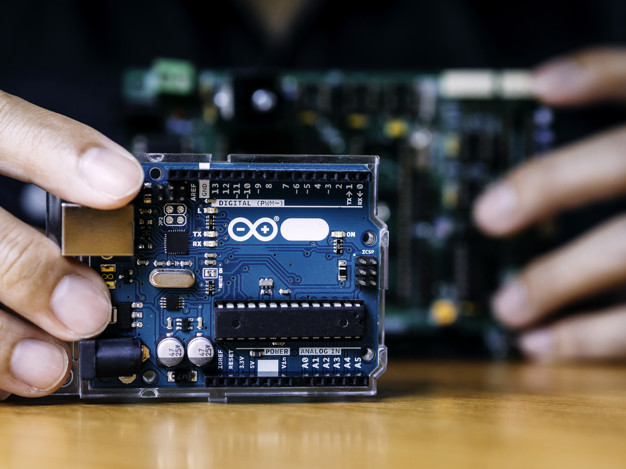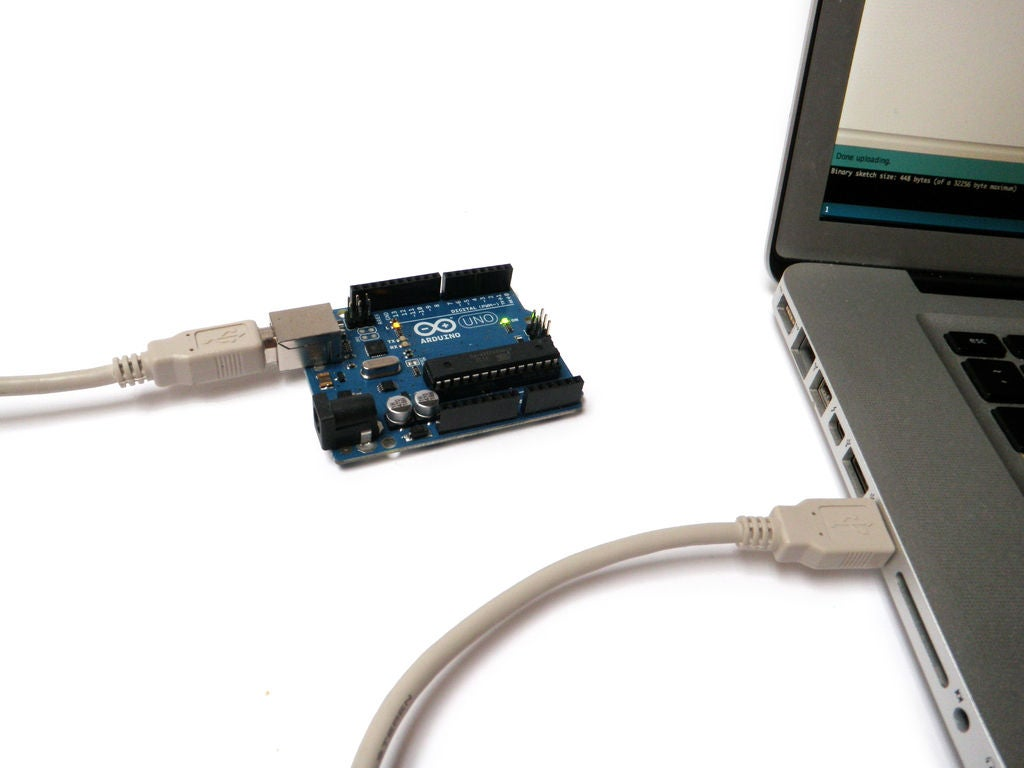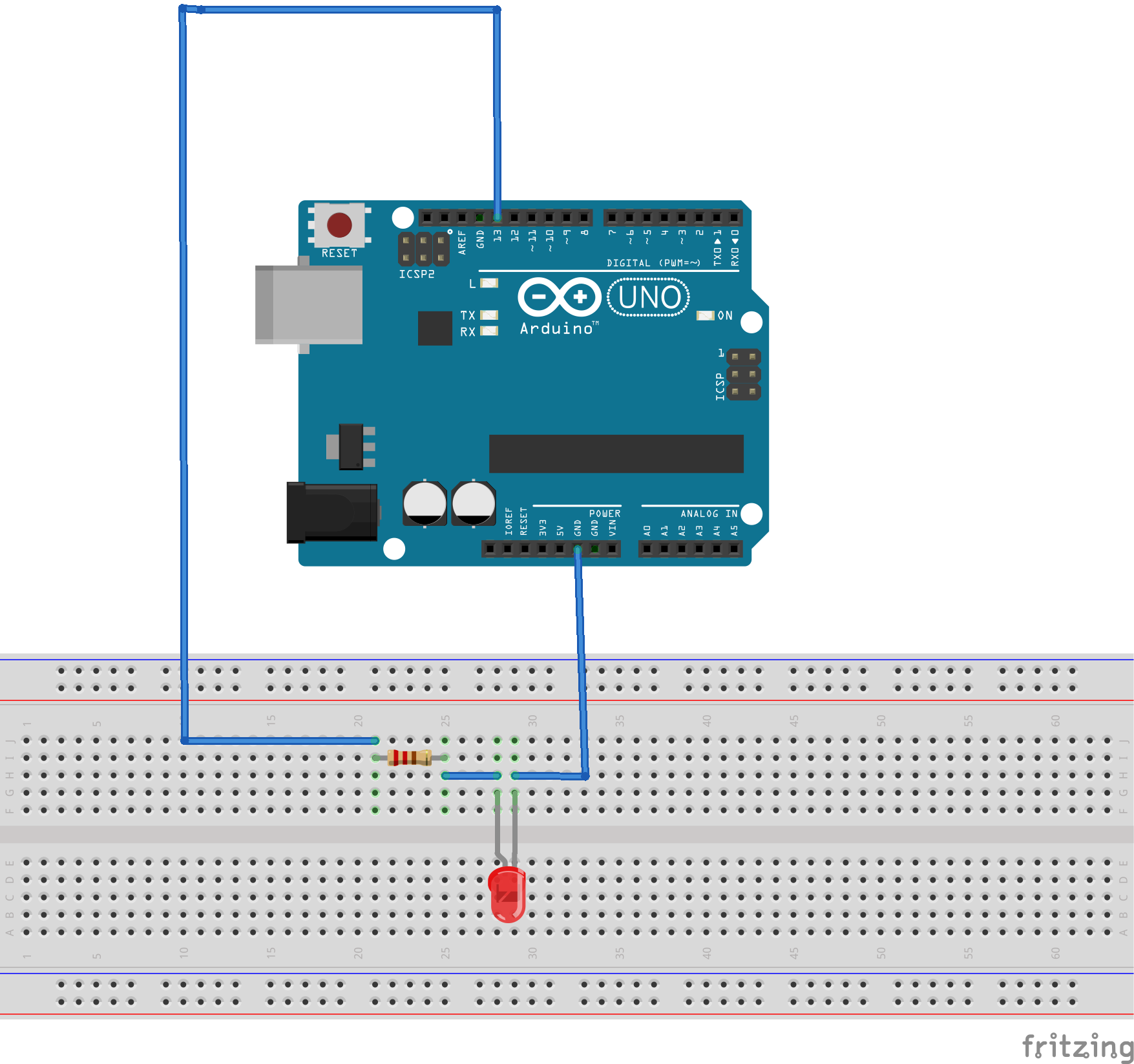# Learn Arduino Basics & How to Get Started

## Getting Started

First, you'll need an Arduino... obviously. However, there are quite a few types of Arduinos to choose from. The most common type for learners is the Arduino Uno which is roughly the size of the palm of your hand. The other Arduino options have different processor speeds, different sizes and different processor speeds so depending on what you need you may choose something different for your project.

However, all Arduinos are programmed the exact same way. They all use the Arduino IDE which can be downloaded here: https://www.arduino.cc/en/main/software

There you'll find a few download options. Just pick the one that best fits your system. Once you've downloaded it, you'll have to go through the install process. You don't need to do anything special for this step so just agree to the license and click next until it installs the default IDE for you.

Once installed and you've got your Arduino with you, you can just plug it into your computer with the USB cable. It should look something like this:There may be small differences if you've got a different type of Arduino; although, all Arduinos will be connected to a PC one way or another.

Now open the Arduino IDE on your computer and make sure that you've got your IDE set up for the right type of Arduino. You can do this by going to Tools > Board and make sure that you have the right board type selected. Again, if your new to this you'll likely be selecting the Arduino UNO option, but you can play around with it if you're unsure.

Then, go to Tools > Ports and make sure that you have the right port selected. This is important because you need the computer to know which USB port the Arduino is connected to. Usually there is only one option so selected that. If there is more than one option try to disconnect the USB cable and see which options change.

Cool! Now you should have everything set up to start writing some code. So lets get started!

## The Code

If you don't know anything about programming that's alright. We'll go over some of the basics here. The Arduino IDE uses a set of C/C++ functions that we're going to go over.

The first thing you'll see when you start a project is this:

``````void setup() {
// put your setup code here, to run once:

}

void loop() {
// put your main code here, to run repeatedly:

}``````

There you've got the setup function and the loop function. The setup function is code that will run once and the loop is code that will run repeatedly. So, when you turn on the Arduino with the code compiled everything in setup will run line-by-line and after the setup runs then everything in the loop function will run line-by-line. After every single line in the loop function runs then it will go back to the top of the loop function and do it all over again.

The Blinking LED is the easiest way to learn about the Arduino. So lets start by adding a variable to the top of the file called ledPin and set it equal to 13. We can do this by typing this:

``````const int ledPin = 13;

void setup() {
// put your setup code here, to run once:

}

void loop() {
// put your main code here, to run repeatedly:

}``````

So what we've done here is set a global variable equal to 13. A global variable is a variable that can be used everywhere. So we can type ledPin inside the setup function and inside the loop function and the program will know that it's equal to 13. We could just type 13 instead, but it's nice to have a single name for the pin that we're going to use.

Cool, so now lets do the next step which will be typing something inside of the setup function. We need to open up the port and set the baud rate. We can do this by typing the following:

``Serial.begin(9600);``

We also need to enable the pin we want to use in the setup function. This will allow us to turn the pin on and off later in the program. We can do this by typing:

``pinMode(ledPin, OUTPUT);``

That takes care of everything we need to do for setup. Now we can just turn the pin on and off. Or, to be more precise we will be setting the pin to either high or low voltage. High voltage is at 5 volts and low voltage is at 0 volts or ground. We can turn the pin high by typing the following:

``digitalWrite(ledPin, HIGH);``

We can also set the pin low with the same function by typing:

``digitalWrite(ledPin, LOW);``

Now the whole program we've written should look like this:

``````const int ledPin = 13;

void setup() {
Serial.begin(9600);
pinMode(ledPin, OUTPUT);
}

void loop() {
digitalWrite(ledPin, HIGH);
digitalWrite(ledPin, LOW);
}``````

So, if we were to connect an LED to pin 13 (which I'll talk more about how to do later) then we would see that the LED stays on. This is because the LED is blinking so fast that our eyes cannot see it blink. If we want the LED to blink more slowly then we can use the delay function. Here is what that would look like:

``````const int ledPin = 13;

void setup() {
Serial.begin(9600);
pinMode(ledPin, OUTPUT);
}

void loop() {
digitalWrite(ledPin, HIGH);
delay(100);
digitalWrite(ledPin, LOW);
delay(100);
}``````

Here we are calling the delay function in two places with a single parameter. The parameter that the function takes is the time of delay and it's in milliseconds.

So now lets make the circuit for a blinking LED.

## The Circuit

To make the circuit simply connect wires as seen in the picture below.This turns the LED on and off because we are switching pin 13 from 5 volts to 0 volts every 100 milliseconds. When its at 5 volts current flows from pin 13 to the ground (GND) pin and when its at 0 volts no current flows and therefore the LED is off.

We can use the digitalWrite function to do more than just turn on and off an LED however. All that command does is change a voltage which gives us a lot of options for some creativity. If we wanted to use the pin to turn on and off a motor or speaker we can do that as well. We can even control how fast the motor turns using PWM.

If you have any questions or you'd like another tutorial let me know in the comments!